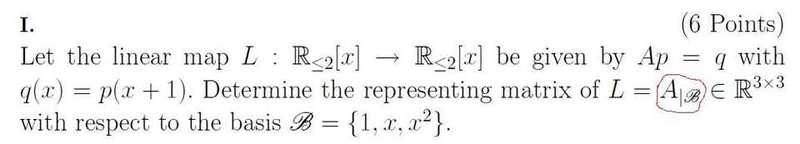# Linear Algebra 2 - Representing Matrix

Sry, this will be the last question^^Its a similiar to the problem i ve postet before. Maybe if i can solve the first problem i can solve this to. But what i dont understand is that notation. I ve circled it with a red line.
Does anyone know what this means?

Thx
Mumba

dx
Homework Helper
Gold Member
It means the representation of L in the basis B = {1, x, x²}. They're just calling that matrix AB

So this A is the representing matrix?
But what does then Ap=q mean? Or how can i calculate L for 1, x and x^2?

dx
Homework Helper
Gold Member
Ap = p(x+1) defines the action of L.

So for p = 1, you get L(1) = x + 1 and so on.

dx
Homework Helper
Gold Member
Actually, it looks like q(x+1) is not intended as multiplication of q with (x+1). The action of L on the function p(x) is given by p(x+1).

So, if p = 1 + x, then L(p) = 1 + (x + 1) = 2 + x.

But so i get for
L(x) = x^2 + x,
L(x^2)=x^3 + x^2

So should i choose a new basis, for example {1+x,x^2,x^3} to get the repr matrix?

dx
Homework Helper
Gold Member
See my post above.

L(x) will be x + 1 instead of x² + x.

L(p)=q(p)=p(x+1)?

wie kommst du da auf L(x)=1+x?
Was hast du denn dann für L(1)?

dx
Homework Helper
Gold Member
Treat a polynomial as the function p(x). The action of L is to take that function and return the function q(x) = p(x+1)

So, if p(x) = 1 + x, then q(x) = p(x + 1) = 1 + x + 1 = x + 2 (i.e. L[1 + x] = 2 + x)

If p(x) = 1, then q(x) = p(x + 1) = 1. (i.e L = 1 )

So i get then
L(1) = 1
L(x) = x+1
L(x^2)=x^2+1

?

dx
Homework Helper
Gold Member
L[x²] = (x+1)² = x² + 2x + 1

and then for
L(1) --> 1 0 0
L(x) --> 1 1 0
L(x^2) --> 1 0 1

So my matrix would be
1 1 1
0 1 0
0 0 1

??

ahh ok so if u change this fpr L(x^2) --> 1 2 1
matrix:
1 1 1
0 1 2
0 0 1

correct? ^^

dx
Homework Helper
Gold Member
Yes, thats correct.

:D:D
cool thx alooooot

Treat a polynomial as the function p(x). The action of L is to take that function and return the function q(x) = p(x+1)

So, if p(x) = 1 + x, then q(x) = p(x + 1) = 1 + x + 1 = x + 2 (i.e. L[1 + x] = 2 + x)

If p(x) = 1, then q(x) = p(x + 1) = 1. (i.e L = 1 )

Should it be like that:

If p(x) =1 then, q(x) = p(x+1) = p(x)+p(1) = 1 + 1 = 2, so L= 2!?

dx
Homework Helper
Gold Member
No, p(x) is a constant function, i.e. it has the same value for any x. So p(x + 1) would still be 1.

hmm, ok
but why ist L(x) = x+1?

--> p(x) = x
if its a constant function and p(x)=x, shouldnt be P(x+1)=x too???

dx
Homework Helper
Gold Member
p(x) = 1 is a constant function, p(x) = x is not!

For a given polynomial p, we get the L[p] by replacing every x with x + 1.

So if p = 1, we do nothing since there is no x, and L = 1

If p = x, we replace x by x + 1, and we get L[x] = x + 1

If p = x2, we replace x by x + 1 to get (x + 1)2, i.e. L[x2] = (x + 1)2 = 1 + 2x + x2

oh man
yes sure thx again ^^